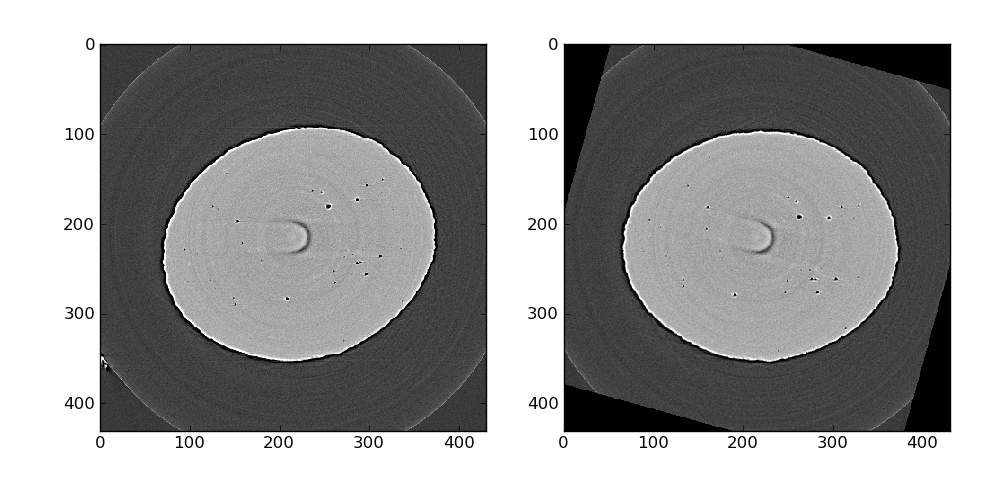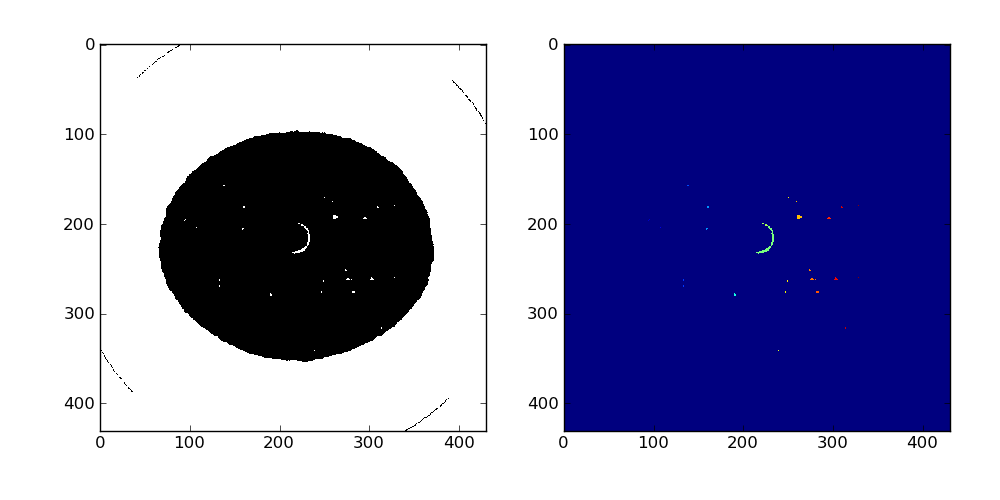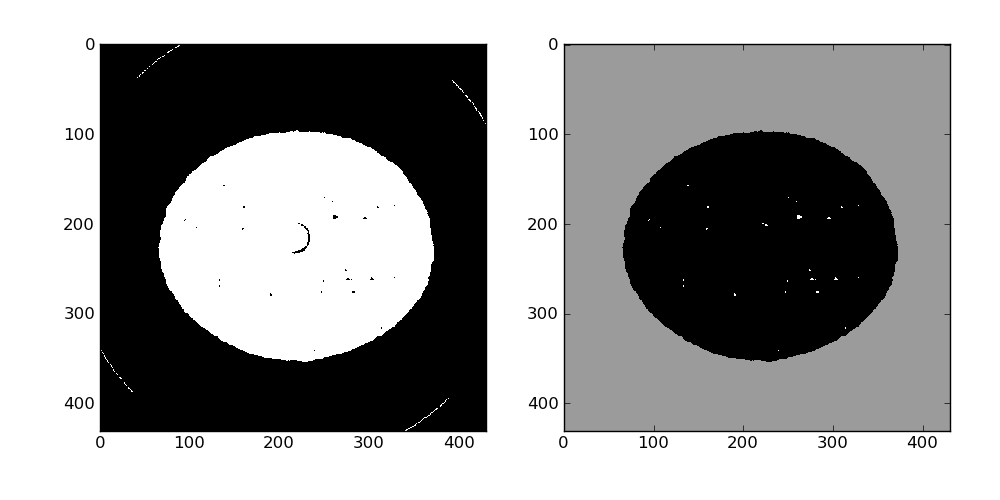# 3d analysis and rendering of damage in a steel tension sample¶

This recipe demonstrate how to analyze the cavities in a steel sample deformed under tension loading.

Get the complete Python source code: `steel_damage_analysis.py`

The 3d data set is a 8 bits binary file with size 341x341x246. First you need to define the path to the 3d volume:

```data_dir = '../../examples/data'
scan_name = 'steel_431x431x246_uint8'
scan_path = os.path.join(data_dir, scan_name + '.raw')
```

```data = HST_read(scan_path, header_size=0)
```

The reconstructed tomographic volume is not align with the cartesian axes, so rotate the volume by 15 degrees:

```data = ndimage.rotate(data, 15.5, axes=(1, 0), reshape=False)
```

The result of these operations can be quickly observed using regular pyplot functions such as imshow. Here we look at slice number 87 where a ring artifact is present. We will take care of those later.The entire volume is binarized using a simple threshold with the value 100:

```data_bin = np.where(np.greater(data, 100), 0, 255).astype(np.uint8)
```

And cavities are labeled using standard scipy tools:

```label_im, nb_labels = ndimage.label(data_bin)
```

3465 labels have been found, the result can be observed on slice 87 using pyplot:We use a simple algorithm to remove ring articfacts (assuming the cavities are convex): the center of mass of each label is compute and if it does not lie inside the label, the corresponding voxels are set to 0. Here 11 rings were removed.

```# simple ring removal artifact
coms = ndimage.measurements.center_of_mass(data_bin / 255, labels=label_im, \
index=range(1, nb_labels + 1))
rings = 0
for i in range(nb_labels):
com_x = round(coms[i])
com_y = round(coms[i])
com_z = round(coms[i])
if not label_im[com_x, com_y, com_z] == i + 1:
print 'likely found a ring artifact at (%d, %d, %d) for label = %d, value is %d' \
% (com_x, com_y, com_z, (i + 1), label_im[com_x, com_y, com_z])
data_bin[label_im == (i + 1)] = 0
rings += 1
print 'removed %d rings artifacts' % rings
```

The outside of the sample needs a little love, it is by far the largest label here so after inverting the labeled image and running a binary closing it can be set to a fixed value (155 here) in the data_bin array.

```print('labeling and using a fixed color around the specimen')
# the outside is by far the largest label here
print('inverting the image so that outside is now 1')
data_inv = 1 - label_im```plt.subplots_adjust(left=0.1, right=0.95, top=0.95, bottom=0.1)